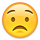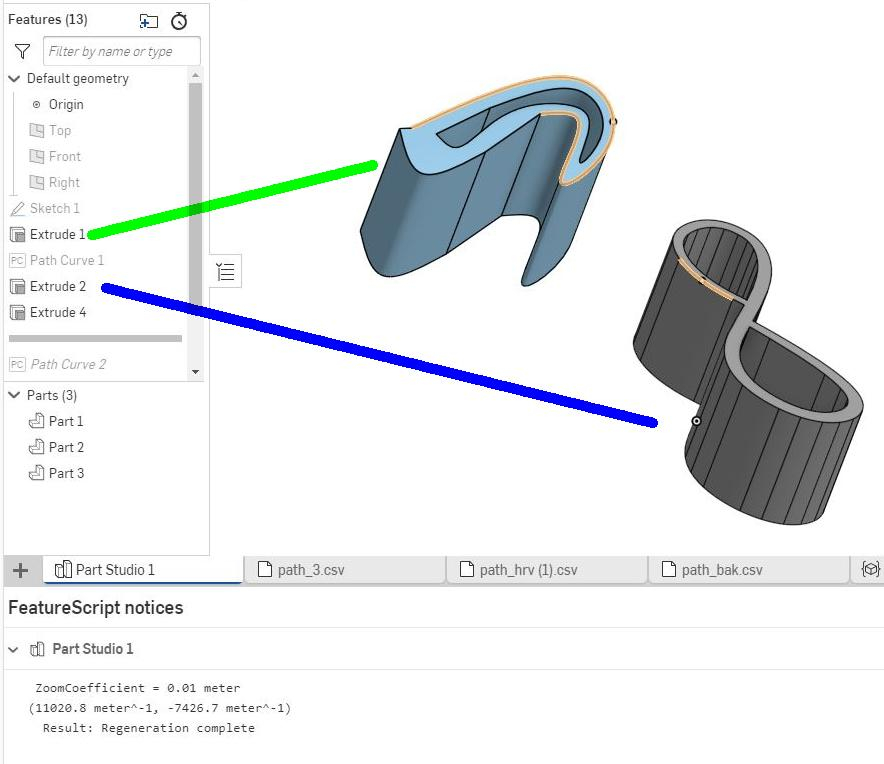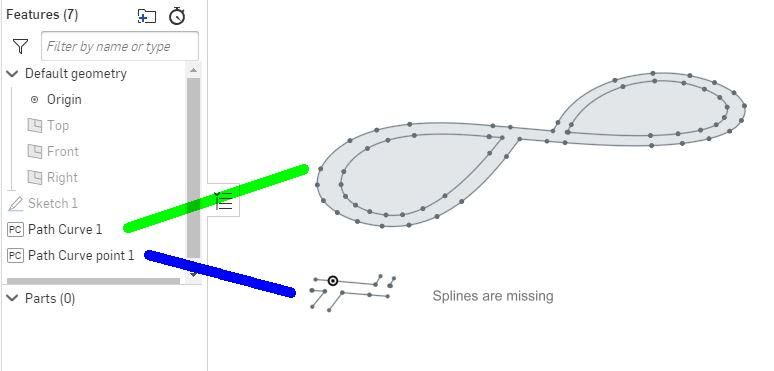Welcome to the Onshape forum! Ask questions and join in the discussions about everything Onshape.

First time visiting? Here are some places to start:
1. Looking for a certain topic? Check out the categories filter or use Search (upper right).
2. Need support? Ask a question to our Community Support category.
3. Please submit support tickets for bugs but you can request improvements in the Product Feedback category.
4. Be respectful, on topic and if you see a problem, Flag it.

If you would like to contact our Community Manager personally, feel free to send a private message or an email.

# Spline with an array

Hello

I need some help with the spline.
I wrote a feature which draw a spline from a csv file
Inside I use this code

```} else if (linetype == "C") {
var P1 = point_courant;
var P2 = vector(aline, -aline) * ZoomCoefficient + splineOffset;
var P3 = vector(aline, -aline) * ZoomCoefficient + splineOffset;
var P4 = vector(aline, -aline) * ZoomCoefficient + splineOffset;

var start = P1;
var end = P4;
var startDerivative = 3 * (P2 - P1);
var endDerivative = 3 * (P4 - P3);

skFitSpline(sketch1, "spline_"~ entityCount*100, {
"points": [
start,
end
],
"startDerivative": startDerivative,
"endDerivative": endDerivative,
});
point_courant = P4;
}```

Unfortunately the display is not the same as with the spline tool
There is lots of "glued splines" but not a single smooth curve
-- In green a spline created with the spline tool : separation are shown only when curve is a line.
-- In blue the one from my featureScript: separation are shown for each points of the splineI changed my way to draw all the connected point at once with this code
1/ first detect the end of point list to draw all at once
```if ((lastlinetype == "C") && (linetype != "C")) {
if (splineopened) {
print("spline_"~ entityCount);
print(" = ");
skFitSpline(sketch1, "spline_"~ entityCount , {
"points": splinePoints,
"startDerivative": startDerivative,
"endDerivative": endDerivative,
});
println(splinePoints);
splinePoints = [];
splineopened = false;
}
}```
2/ and instead of drawing the curve, I memorize the points in a list

```} else if (linetype == "C") {
var P1 = point_courant;
var P2 = vector(aline, -aline) * ZoomCoefficient + splineOffset;
var P3 = vector(aline, -aline) * ZoomCoefficient + splineOffset;
var P4 = vector(aline, -aline) * ZoomCoefficient + splineOffset;

var start = P1;
var end = P4;
endDerivative = 3 * (P4 - P3);
splinePoints = append(splinePoints, start);
splinePoints = append(splinePoints, end);
if(splineopened == false) {
startDerivative = 3 * (P2 - P1);
}
splineopened = true;
point_courant = P4;
}```
But it does not work!!!And I don't understand what is wrong

Can you help ?
Tagged: